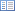Code of Federal Regulations (Last Updated: April 25, 2019)Title 40 - Protection of EnvironmentCHAPTER I — ENVIRONMENTAL PROTECTION AGENCYSUBCHAPTER C — AIR PROGRAMSPart 60 - STANDARDS OF PERFORMANCE FOR NEW STATIONARY SOURCES

# Appendix C to Part 60—Determination of Emission Rate Change

• ## 1. Introduction

1.1 The following method shall be used to determine whether a physical or operational change to an existing facility resulted in an increase in the emission rate to the atmosphere. The method used is the Student's t test, commonly used to make inferences from small samples.

## 2. Data

2.1 Each emission test shall consist of n runs (usually three) which produce n emission rates. Thus two sets of emission rates are generated, one before and one after the change, the two sets being of equal size.

2.2 When using manual emission tests, except as provided in §60.8(b) of this part, the reference methods of appendix A to this part shall be used in accordance with the procedures specified in the applicable subpart both before and after the change to obtain the data.

2.3 When using continuous monitors, the facility shall be operated as if a manual emission test were being performed. Valid data using the averaging time which would be required if a manual emission test were being conducted shall be used.

## 3. Procedure

3.1 Subscripts a and b denote prechange and postchange respectively.

3.2 Calculate the arithmetic mean emission rate, E, for each set of data using Equation 1.Ei = Emission rate for the i th run.

n = number of runs.

3.3 Calculate the sample variance, S2, for each set of data using Equation 2.3.4 Calculate the pooled estimate, Sp, using Equation 3.3.5 Calculate the test statistic, t, using Equation 4.## 4. Results

4.1 If Eb>,Ea and t>t′, where t′ is the critical value of t obtained from Table 1, then with 95% confidence the difference between Eb and Ea is significant, and an increase in emission rate to the atmosphere has occurred.

For greater than 8 degrees of freedom, see any standard statistical handbook or text.

5.1 Assume the two performance tests produced the following set of data:

5.2 Using Equation 1—

5.3 Using Equation 2—

Sa2 = (100−102)2 + (95−102)2 + (110−102)2/3−1 = 58.5

Sb2 = (115−120)2 + (120−120)2 + (125−120)2/3−1 = 25

5.4 Using Equation 3—

Sp = [(3 − 1)(58.5) + (3 + 1)(25) / 3 + 3 − 2] 12 = 6.46

5.5 Using Equation 4—